# 17 4 Calculating Heats of Reaction CALCULATING HEATS

• Slides: 24
Download presentation17. 4 Calculating Heats of ReactionCALCULATING HEATS OF REACTION OBJECTIVES • Apply Hess’s law of heat summation to find enthalpy changes for chemical and physical processes • Calculate formation enthalpy changes using standard heats of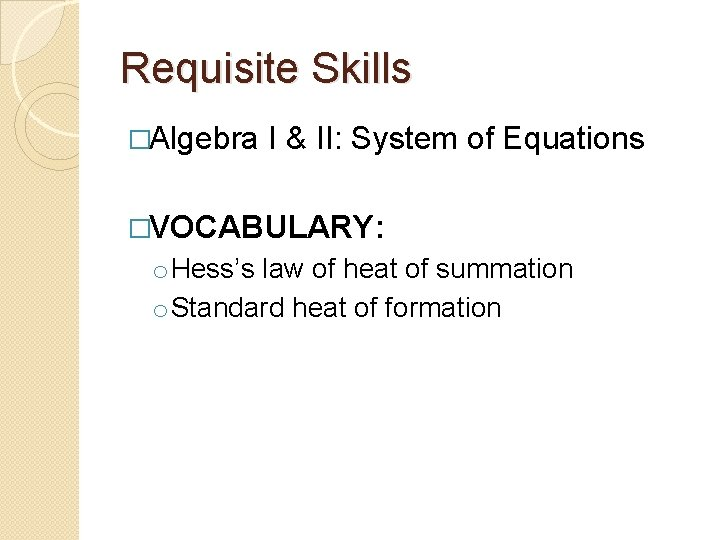Requisite Skills �Algebra I & II: System of Equations �VOCABULARY: o Hess’s law of heat of summation o Standard heat of formation. . . manipulate and combine algebraic equations. In algebra I, you learned that you can combine the like terms from two equations to make a third equation. 2 x + 6 y = 16 y = 2 x + 12 + 2 x + 7 y = 2 x + 28 7 y = 28 y=4 4 = 2 x + 12 x = -4 If you do this right, this allows you to solve for one of the variables by eliminating the other one. Once you know of the variables, you can plug it in and solve for the other variable. 4 - 12 = 2 x + 12 -8 = 2 x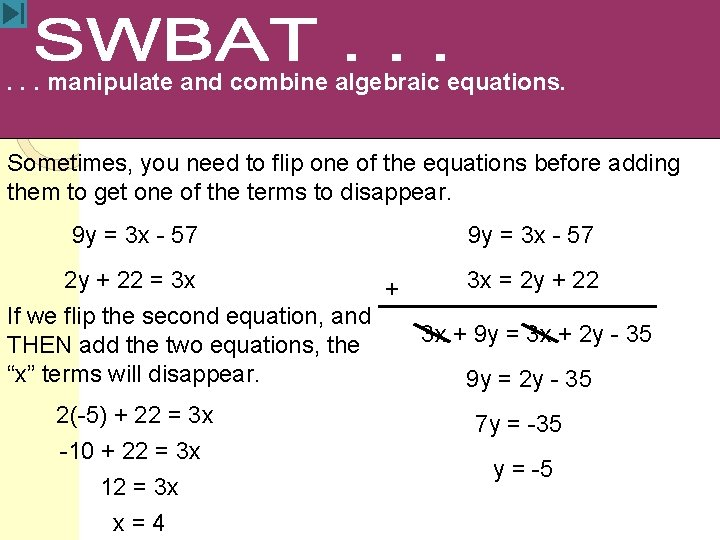. . . manipulate and combine algebraic equations. Sometimes, you need to flip one of the equations before adding them to get one of the terms to disappear. 9 y = 3 x - 57 2 y + 22 = 3 x 3 x = 2 y + 22 + If we flip the second equation, and 3 x + 9 y = 3 x + 2 y - 35 THEN add the two equations, the “x” terms will disappear. 9 y = 2 y - 35 2(-5) + 22 = 3 x 7 y = -35 -10 + 22 = 3 x y = -5 12 = 3 x x=4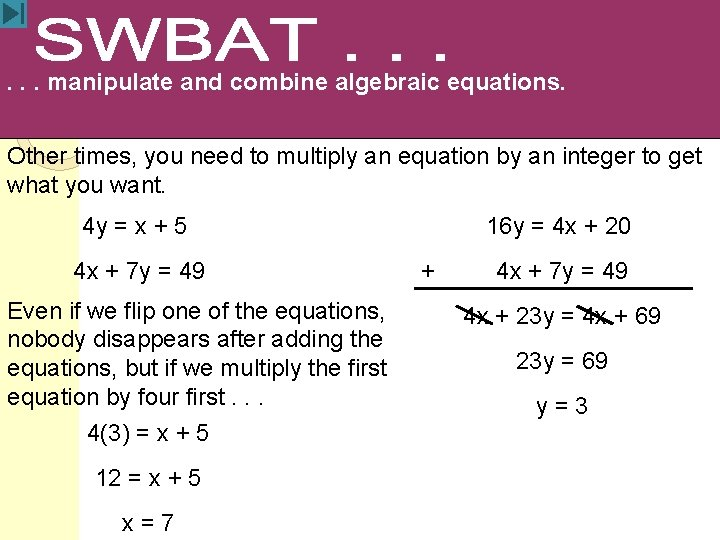. . . manipulate and combine algebraic equations. Other times, you need to multiply an equation by an integer to get what you want. 4 y = x + 5 4 x + 7 y = 49 Even if we flip one of the equations, nobody disappears after adding the equations, but if we multiply the first equation by four first. . . 4(3) = x + 5 12 = x + 5 x=7 16 y = 4 x + 20 + 4 x + 7 y = 49 4 x + 23 y = 4 x + 69 23 y = 69 y=3. . . manipulate and combine algebraic equations. Sometimes, you can use these old algebra tricks with chemical equations to solve for unknowns. . .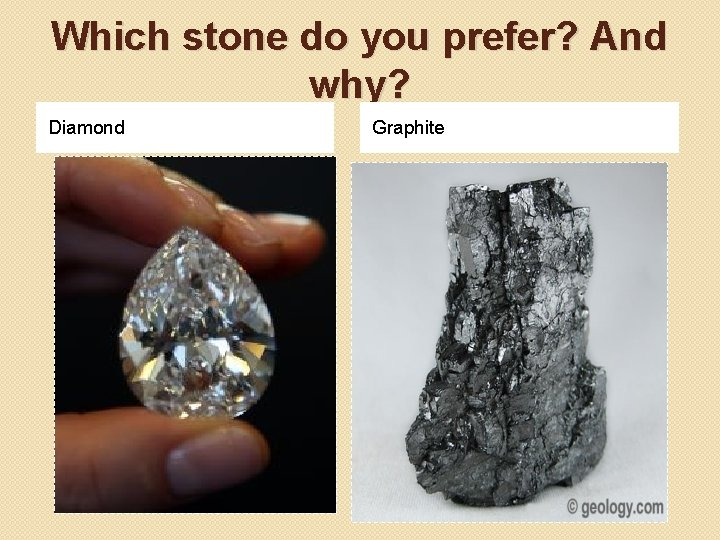Which stone do you prefer? And why? Diamond GraphiteWould you like Diamond to be converted into Graphite? �Such conversion or reaction will take millions of years to complete �And chemists are curious to know the enthalpy changes for the conversion of diamond to graphite. �Should they wait million of years when the reaction will be completed to get the data? Or �What do you recommend they should do? So that the enthalpy changes data would be available ASAP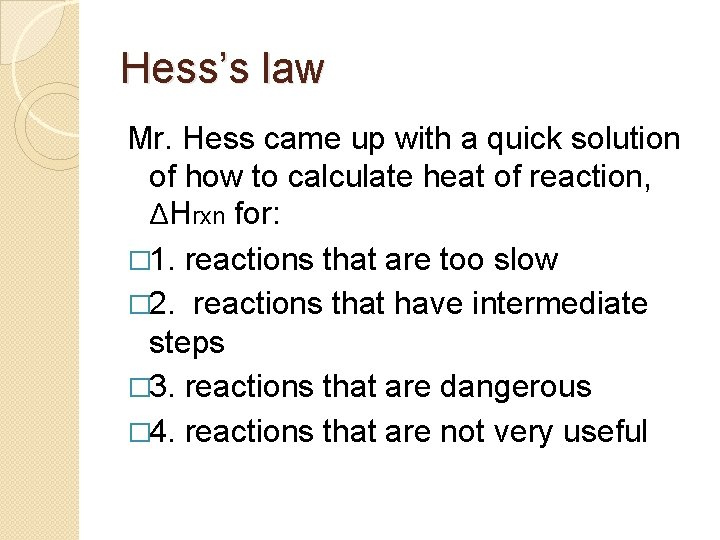Hess’s law Mr. Hess came up with a quick solution of how to calculate heat of reaction, ΔHrxn for: � 1. reactions that are too slow � 2. reactions that have intermediate steps � 3. reactions that are dangerous � 4. reactions that are not very usefulHess’s law Heat of Summation �States that if you add to two or more thermochemical equations to give final equation, then you can also add the heat of reaction to give the final heat of reaction �Hess’s law allows us to determine the heat of reaction indirectly.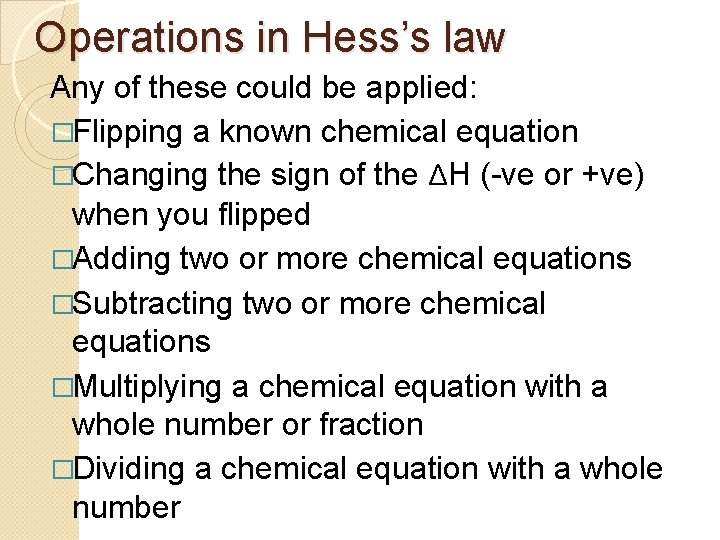Operations in Hess’s law Any of these could be applied: �Flipping a known chemical equation �Changing the sign of the ΔH (-ve or +ve) when you flipped �Adding two or more chemical equations �Subtracting two or more chemical equations �Multiplying a chemical equation with a whole number or fraction �Dividing a chemical equation with a whole number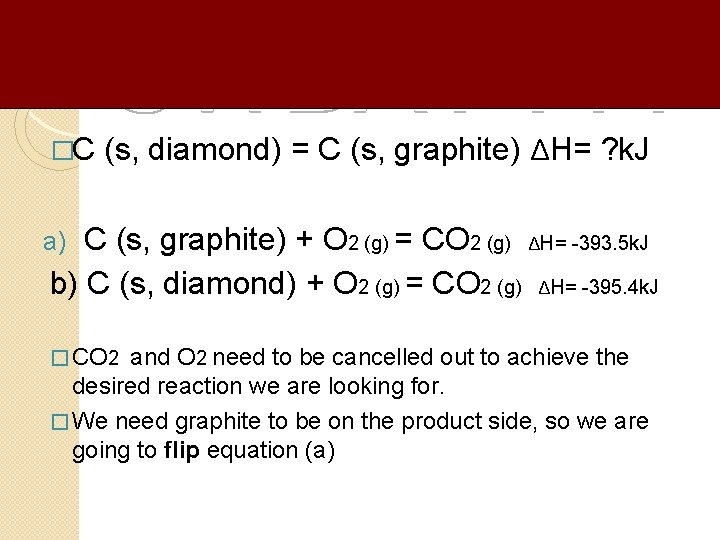�C (s, diamond) = C (s, graphite) ΔH= ? k. J C (s, graphite) + O 2 (g) = CO 2 (g) ΔH= -393. 5 k. J b) C (s, diamond) + O 2 (g) = CO 2 (g) ΔH= -395. 4 k. J a) � CO 2 and O 2 need to be cancelled out to achieve the desired reaction we are looking for. � We need graphite to be on the product side, so we are going to flip equation (a)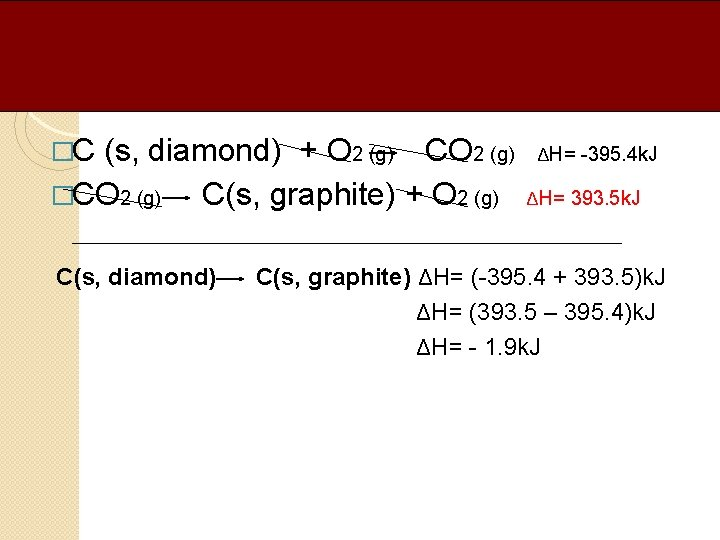�C (s, diamond) + O 2 (g) CO 2 (g) �CO 2 (g) C(s, graphite) + O 2 (g) C(s, diamond) ΔH= -395. 4 k. J ΔH= 393. 5 k. J C(s, graphite) ΔH= (-395. 4 + 393. 5)k. J ΔH= (393. 5 – 395. 4)k. J ΔH= - 1. 9 k. J. . . use Hess’ law to determine DHrxn Hess’s law allows us to figure out DHrxn for a reaction without ever having to make the reaction happen in real life. HLEx 1: Imagine that experiments tell you the following: Sn(s) + Cl 2(g) Sn. Cl 2(s) DH = -325. 1 k. J Sn. Cl 2(s) + Cl 2(g) Sn. Cl 4(l) DH = -186. 2 k. J If you add the two equations above, you get the following: Sn(s) + Sn. Cl 2(s) + 2 Cl 2(g) Sn. Cl 2(s) + Sn. Cl 4(l) Just as with normal, algebraic equations, when the same term appears on the left and right, it can be crossed out. . . yielding. . . Sn(s) + 2 Cl 2(g) Sn. Cl 4(l) DH = ? k. J. . . use Hess’ law to determine DHrxn Now here’s the real magic. Hess’s law says that you can also add the DH’s for the reactions to get the DH for the final reaction. Sn(s) + Cl 2(g) Sn. Cl 2(s) + Cl 2(g) Sn. Cl 4(l) DH = -325. 1 k. J DH = -186. 2 k. J (-325. 11 k. J) + (-186. 2 k. J) = -511. 3 k. J The sneaky miracle here is that we figured this out without ever having to make tin metal and chlorine gas react to form tin (IV) chloride in real life. Sn(s) + 2 Cl 2(g) Sn. Cl 4(l) DH==-511. 3 ? k. J DH. . . use Hess’ law to determine DHrxn That was a very simple usage of Hess’ law. We didn’t have to manipulate any equations before adding them. Let’s try a harder problem.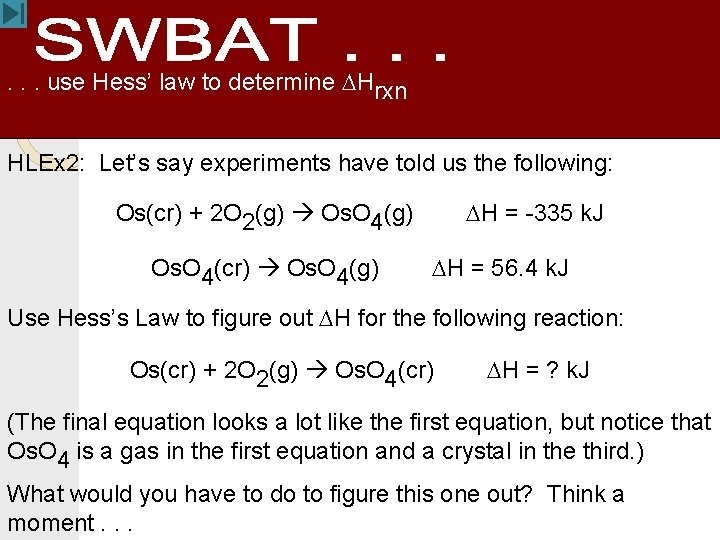. . . use Hess’ law to determine DHrxn HLEx 2: Let’s say experiments have told us the following: DH = -335 k. J Os(cr) + 2 O 2(g) Os. O 4(g) Os. O 4(cr) Os. O 4(g) DH = 56. 4 k. J Use Hess’s Law to figure out DH for the following reaction: Os(cr) + 2 O 2(g) Os. O 4(cr) DH = ? k. J (The final equation looks a lot like the first equation, but notice that Os. O 4 is a gas in the first equation and a crystal in the third. ) What would you have to do to figure this one out? Think a moment. . .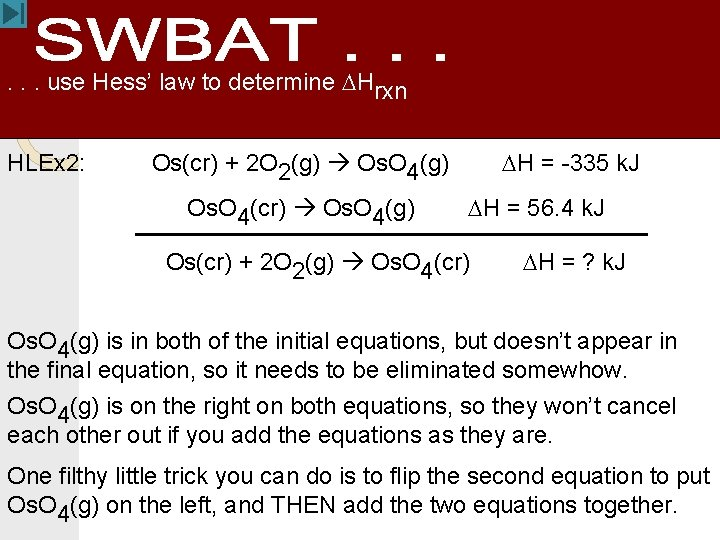. . . use Hess’ law to determine DHrxn HLEx 2: DH = -335 k. J Os(cr) + 2 O 2(g) Os. O 4(g) Os. O 4(cr) Os. O 4(g) DH = 56. 4 k. J Os(cr) + 2 O 2(g) Os. O 4(cr) DH = ? k. J Os. O 4(g) is in both of the initial equations, but doesn’t appear in the final equation, so it needs to be eliminated somewhow. Os. O 4(g) is on the right on both equations, so they won’t cancel each other out if you add the equations as they are. One filthy little trick you can do is to flip the second equation to put Os. O 4(g) on the left, and THEN add the two equations together.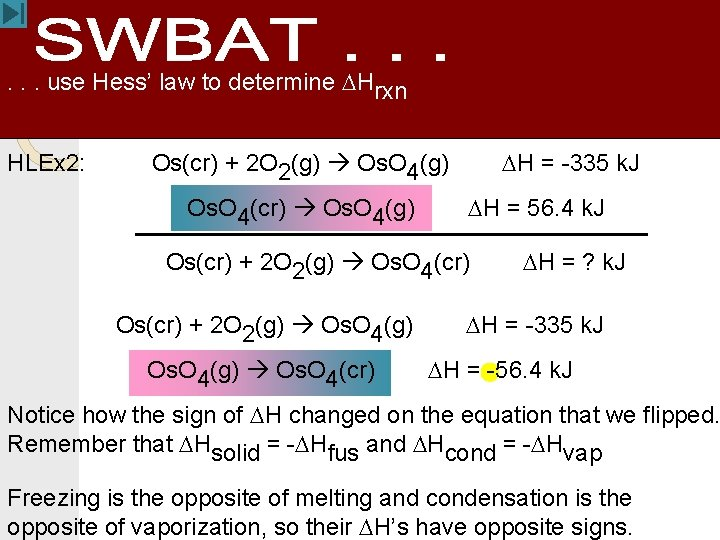. . . use Hess’ law to determine DHrxn HLEx 2: DH = -335 k. J Os(cr) + 2 O 2(g) Os. O 4(g) Os. O 4(cr) Os. O 4(g) DH = 56. 4 k. J Os(cr) + 2 O 2(g) Os. O 4(cr) Os(cr) + 2 O 2(g) Os. O 4(g) Os. O 4(cr) DH = ? k. J DH = -335 k. J DH = -56. 4 k. J Notice how the sign of DH changed on the equation that we flipped. Remember that DHsolid = -DHfus and DHcond = -DHvap Freezing is the opposite of melting and condensation is the opposite of vaporization, so their DH’s have opposite signs.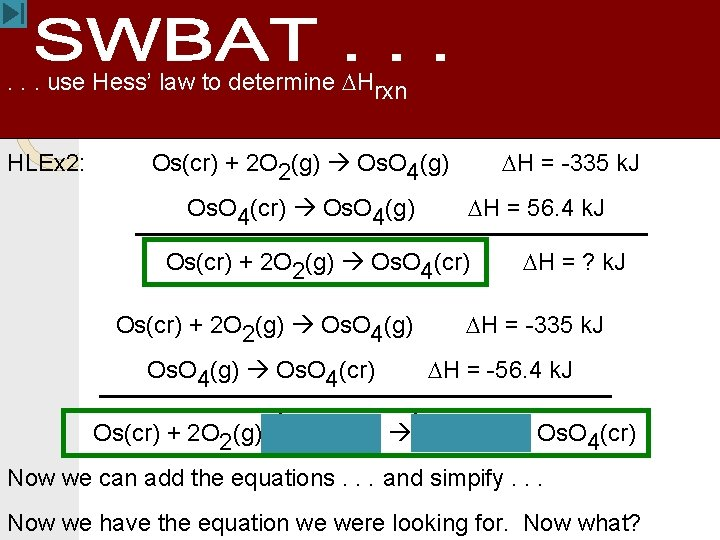. . . use Hess’ law to determine DHrxn HLEx 2: DH = -335 k. J Os(cr) + 2 O 2(g) Os. O 4(g) Os. O 4(cr) Os. O 4(g) DH = 56. 4 k. J Os(cr) + 2 O 2(g) Os. O 4(cr) Os(cr) + 2 O 2(g) Os. O 4(g) Os. O 4(cr) DH = ? k. J DH = -335 k. J DH = -56. 4 k. J Os(cr) + 2 O 2(g) + Os. O 4(g) + Os. O 4(cr) Now we can add the equations. . . and simpify. . . Now we have the equation we were looking for. Now what?. . . use Hess’ law to determine DHrxn HLEx 2: DH = -335 k. J Os(cr) + 2 O 2(g) Os. O 4(g) DH = 56. 4 k. J Os. O 4(cr) Os. O 4(g) Os(cr) + 2 O 2(g) Os. O 4(cr) DH = -335 k. J Os(cr) + 2 O 2(g) Os. O 4(g) Os. O 4(cr) DH = ? k. J DH = -56. 4 k. J Os(cr) + 2 O 2(g) + Os. O 4(g) + Os. O 4(cr) Add the DH’s to get the DH for the final equation. DH = -335 k. J + (-56. 4 k. J) = -391. 4 k. J = DH. . . use standard heats of formation to determine DHrxn [Section under construction]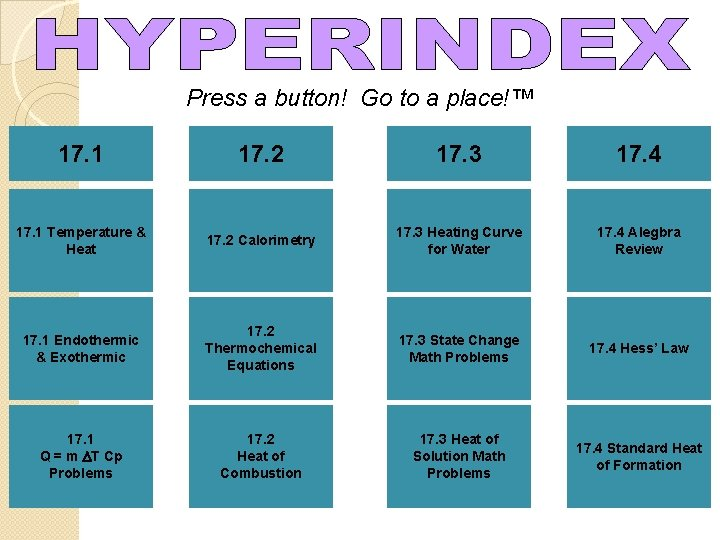Press a button! Go to a place!™ 17. 1 17. 2 17. 3 17. 4 17. 1 Temperature & Heat 17. 2 Calorimetry 17. 3 Heating Curve for Water 17. 4 Alegbra Review 17. 1 Endothermic & Exothermic 17. 2 Thermochemical Equations 17. 3 State Change Math Problems 17. 4 Hess’ Law 17. 1 Q = m DT Cp Problems 17. 2 Heat of Combustion 17. 3 Heat of Solution Math Problems 17. 4 Standard Heat of Formation